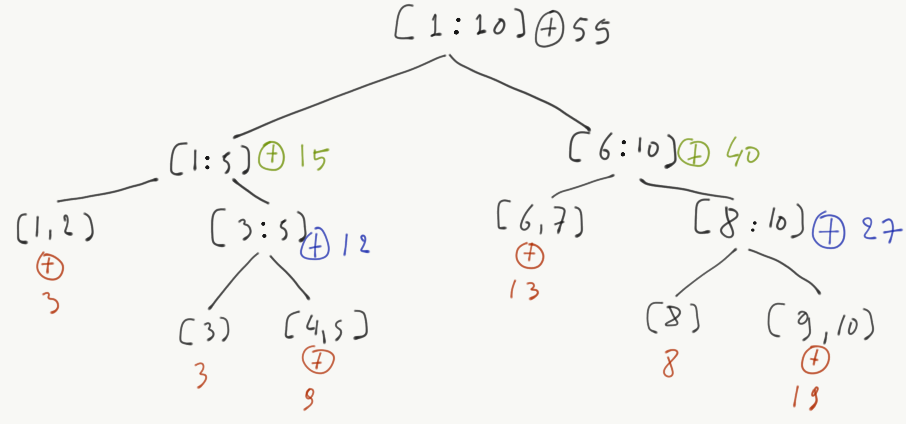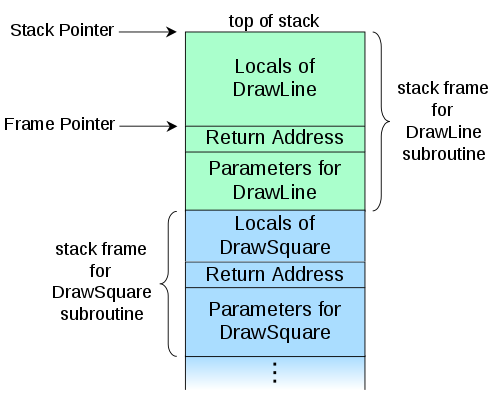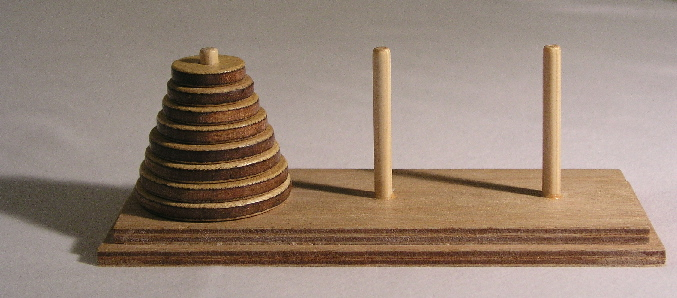# Recursion

## The greatest common divisor problem

The greatest common divisor (GCD) of two integers is the largest positive integer that divides them cleanly.

For example, given $$p = 32$$ and $$q = 24$$, their GCD is 8, even though 2 and 4 are also dividing them cleanly

Q: How can we compute it?

One typical way of computing the GCD is Euclid’s algorithm:

$gcd(p, q) = \begin{cases} p & \text{if } q = 0 \\ gcd(q, p \bmod q), & \text{otherwise} \end{cases}$

## Converting to code

$gcd(p, q) = \begin{cases} p & \text{if } q = 0 \\ gcd(q, p \bmod q), & \text{otherwise} \end{cases}$

Let’s try to convert GCD into code:

def gcd(p, q):
if q == 0:
return p
return gcd(q, p % q)

Let’s try to call it on some examples

print gcd(32, 24)
print gcd(128, 256)
print gcd(10021, 1342)
print gcd(1007, 143)
## 8
## 128
## 11
## 1

## How is gcd evaluated?

We modify gcd to print its arguments

def gcd(p, q):
if q > 0:
mod = p % q
print "p=%d, q=%d, mod=%d" % (p, q, mod)
if q == 0:
return p
return gcd(q, mod)

print gcd(10021, 1342)

The output we get is:

## p=10021, q=1342, mod=627
## p=1342, q=627, mod=88
## p=627, q=88, mod=11
## p=88, q=11, mod=0
## 11

## Recursion

Recursion is a problem solving method where the solution to a problem depends on solutions to smaller instances of the same problem.

In practice, recursive functions follow this pattern:

# Pseudocode
def recursive(args):
if simple_case is True:
return simple_case_result
else:
recursive(simplified(args))

Recursion is everywhere in CS:

• Algorithms
• Data types: List = EmptyList | Cons Item List
• Acronyms: GNU means GNU is Not Unix

## Types of recursion

• Linear recursion: Only one recursive call in function body, e.g. gcd
• Binary recursion: Two recursive calls
• Multiple recursion: Multiple recursive calls

Binary recursion is typical with divide-and-conquer algorithms: the problem is split in two equally sized sub-parts and the solution is recursively computed by aggregating the results of the sub-parts.

Suppose we have an array

a = [1,2,3,4,5,6,7,8]

how can we compute the sum of its elements recursively?

def sum_dc(a):
if len(a) == 1:
return a
if len(a) == 2:
return a + a

mid = len(a) / 2
left = a[0:mid]
right = a[mid:len(a)]
return sum_dc(left) + sum_dc(right)

Of course, this only works because + (addition) is commutitative. It wouldn’t work with, e.g. ‘/’

## How is sum_dc evaluated?Recursive divide and conquer array addition

This is an example of a recursive evaluation tree

## Recursion and the stack

Q: What does the following code do?

def foo(i):
if i == 1000:
return "Done"
return foo(i + 1)

foo(0)
>>> foo(1000)
'Done'
>>> foo(100)
'Done'
>>> foo(0)
[...]
File "<stdin>", line 4, in foo
File "<stdin>", line 4, in foo
File "<stdin>", line 4, in foo
File "<stdin>", line 4, in foo
File "<stdin>", line 4, in foo
RuntimeError: maximum recursion depth exceeded

One typical problem related with recursive algorithms is stack overflow errors!

## What is a call stack?

For the following program

def DrawSquare(p, length):
DrawLine(p.x, p.y, p.x + length, p.y)

When DrawLine is invoked, the computer must know where it will return when doneA call stack

## Tail recursion

If the recursive call is the last statement in a function, then we call this a tail recursion.

To avoid the problem of stack overflows, some languages (not Python) implement an optimization called tail call elimination. This would convert the following code

def foo(i):
if i == 1000:
return "Done"
return foo(i + 1)

into

def foo_opt(i):
while i < 1000:
pass
return "Done"

## Fun with recursion: Towers of HannoiTowers of Hanoi

Move all disks to the right most peg, observing the following rules:

1. Only one disk can be moved at a time.
2. The upper disk from one of the stacks is placed on top of another stack
3. No disk may be placed on top of a smaller disk

## Solution in Python

def hanoi(n, source, tmp, target):
if n == 0:
return

# move tower of size n - 1 to helper
hanoi(n - 1, source, target, tmp)
print "Move disk %d from %s to %s" %(n, source, target)
# move tower of size n-1 from helper to target
hanoi(n - 1, tmp, source, target)

Here is the intuition:

• Move a tower of height-1 to an temp pole, using the final pole.
• Move the remaining disk to the final pole.
• Move the tower of height-1 from the intermediate pole to the final pole using the original pole.

For a visual solution, have a look here